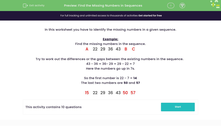# Find the Missing Numbers in Sequences

In this worksheet, students will learn about number sequences by identifying the pattern and finding the missing numbers.Key stage:  KS 2

Curriculum topic:   Number: Number and Place Value

Curriculum subtopic:   Solve Number Problems to 1000

Popular topics:   Ordering Numbers worksheets

Difficulty level:#### Worksheet Overview

In this activity, we will learn to identify the missing numbers in a given sequence.

Look at this sequence.

 15 18 21 24 27 30 33

It goes up in steps of 3.

So, the rule for this sequence is 'add 3'.

Let's look at another sequence. This time some numbers are missing.

 A 22 29 36 43 B C

To find the missing numbers, we first work out the differences between the existing numbers in the sequence.

The difference between 22 and 29 is 7.

29 - 22 = 7

The difference between 36 and 43 is also 7.

43 - 36 = 7

So, we know that the numbers in this sequence go up in 7s.

We can find the first missing number by counting back 7 from 22.

22 - 7 = 15

Then, we can find the second and third missing numbers by counting on in steps of 7.

43 + 7 = 50

50 + 7 = 57

The missing numbers are 15, 50 and 57.

 15 22 29 36 43 50 57

Now let's try an example.

Example:

Find the missing numbers in the sequence.

 A 17 22 27 32 B C

22 - 7 = 5

27 - 22 = 5

32 - 27 = 5

This sequence is counting in 5s.

So the first missing number is 17 - 5 = 12.

The last two numbers are 37 and 42.

 12 17 22 27 32 37 42

Now it's your turn to work out some missing numbers in sequences.

How do you feel about that?### What is EdPlace?

We're your National Curriculum aligned online education content provider helping each child succeed in English, maths and science from year 1 to GCSE. With an EdPlace account you’ll be able to track and measure progress, helping each child achieve their best. We build confidence and attainment by personalising each child’s learning at a level that suits them.

Get started